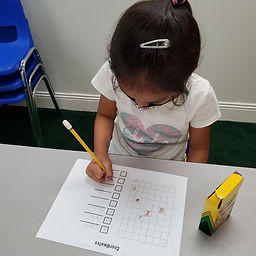## Ms. Raejean

### Target 1​

###### Lesson Type:

Continuation

Geometry

:

Coordinates

Use coordinates (the intersection of a row and column) to indicate the location of an object on a grid.

###### 1:

Understand that in a coordinate, the first number indicates right and left (column) placement and the second up and down (row) placement.

###### 2:

Identify a row and a column.

###### 3:

Use visual tracking to accurately find the intersection point of a row and column.

###### 4:

Explain that coordinates are used so that exact same location can be found by multiple people.

2nd

###### Vocabulary:

Coordinates, Rows, Columns

Activities:

• We reviewed coordinates and how to locate objects on a grid. Students made their own grid of objects and recorded their corresponding coordinates.### Home Exploration

###### Guiding Questions:## Absent Students:

### Target 2

:

###### 1:

Use understandings of addition and subtraction to solve problems where one addend or subtrahend is given and the sum or difference to solve for the unknown number.

###### 2:

Understand the inverse relationship between addition and subtraction.

2nd

###### Vocabulary:

Variables, Fact Families

Activities:

• We used "fact families" to solve equations with variables to determine whether we needed to add or subtract to find the unknown number. Students wrote the known numbers and unknown number (?) on a triangle to represent the fact family. Addition and subtraction symbols were added to the fact family triangle to help us figure out what operation to use. Ex. ? - 16 = 11. Since this is a subtraction equation, the unknown number (?) is the largest number. On the triangle, the unknown number (?) is on the top, 11 and 16 are on the bottom with the addition (+) symbol in between. This tells us that 11 and 16 need to be added to find the unknown number (?).
• We added and subtracted using a hundreds chart, but it would have helped to use manipulatives such as counters or cubes to add and subtract.### Home Exploration

###### Guiding Questions:### Target 3

:

###### Vocabulary:

Activities:### Home Exploration